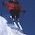## Friday, February 19, 2010

### Desperately seeking a smaller Hilbert space

Following up on an earlier post (with a great cartoon!) How big a Hilbert space do you need?, I have been trying to teach myself some of the basics of Schmidt decompositions of bipartite quantum states (before moving onto multi-particle states). A few things I learnt:

Schmidt decomposition is a restatement of Singular Value Decomposition in a different context.

The number of Schmidt coefficients in the Schmidt decomposition is the Schmidt rank.

The eigenstates of the reduced density matrix on one subsystem are the states in the Schmidt decomposition.

I have a basic question that hopefully some of my quantum information readers can answer.

For pure bipartite states can the minimal value of the Schmidt rank be related to the entanglement of formation (von Neumann entropy of the reduced density matrix)?

The Schmidt decomposition is unique if all of the expansion coefficients are non-zero and non-degenerate. But if some are zero the expansion is not unique. Is there a way to find the shortest possible expansion?

If so, this seems it could be useful when dealing with specific quantum states for which one is trying to find the shortest possible Schmidt decomposition which is "close" to the true state. This is what a lot of quantum chemistry is about: finding the best orbitals and the shortest expansion of Slater determinants.

#### 1 comment:

1."For pure bipartite states can the minimal value of the Schmidt rank be related to the entanglement of formation (von Neumann entropy of the reduced density matrix)? "

Yes. The entropy of one half of a quantum system is always less than the log base 2 of the Schmidt rank. This follows because the entropy is -\sum_i p_i log p_i where p_i=lambda_i^2 and \lambda_i are the Schmidt coefficients. Maximum entropy over k non-zero p_i occurs when each is 1/k and is equal to log of k. So entropy <= log k. (Some terminology: note that "entanglement of formation" = "entropy of entanglement" for pure states, but the concept of "entanglement of formation" also holds for mixed states.)

Not sure what you mean by "minimal" Schmidt rank, though.

"The Schmidt decomposition is unique if all of the expansion coefficients are non-zero and non-degenerate. But if some are zero the expansion is not unique. Is there a way to find the shortest possible expansion?"

Not sure what you mean by this. If you have non-zero Schmidt coefficients, then this just means that your Schmidt decomposition will not include a sum over the entire dimension of your space.

But what you are hinting at is true: you can take the lowest Schmidt coefficients and throw them away and have a pretty good description of the state. This is at the heart of the idea behind recent matrix product algorithms and DMRG.